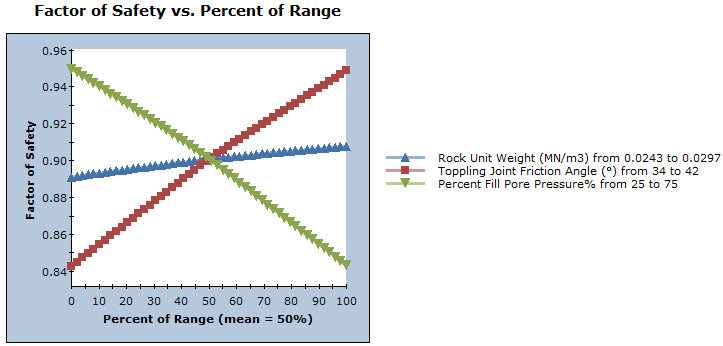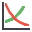# Sensitivity Analysis

The effect of uncertainty or variability in the values of input parameters can be explored using a Sensitivity analysis. In a Sensitivity analysis, model parameters selected by the user are varied across a range of values and the effect on the Factor of Safety is observed. This helps to identify the parameters that have the most effect on the slope stability.

The results of a Sensitivity analysis are displayed on a sensitivity plot in the Sensitivity View, which plots the Factor of Safety against percent change in the input variable(s), as shown in the figure below.On a sensitivity plot, the gradient of a curve for a parameter indicates the effect that parameter has on the Factor of Safety. Steeper (rising or falling) curves indicate greater influence on the Factor of Safety. A relatively "flat" curve indicates that a variable has little effect on the Factor of Safety. A completely flat curve indicates that the variable has NO effect on the Factor of Safety (within the specified range of the variable).

To carry out a Sensitivity analysis with RocTopple:

1. Select Sensitivityon the toolbar or Analysis menu to open the Sensitivity Analysis Input dialog.2. Click the Add button for the number of variables you want to plot (e.g., to plot three variables, click the button three times).
3. Default variables are automatically loaded. To choose the variables you want to plot, click the default variable name and select the variable in the drop-down list. Do this for each variable.
1. Using the From and To input edit boxes, enter a range within which you want each parameter to vary.
2. To display the current input values (i.e., the mean values from the main input data dialogs (Input Data, Support, Loading, Water Pressure) select the Show current project input values check box. This is useful as a guide for defining your range of values for each parameter.
3. When you are done entering parameters, click the Plot button.
A sensitivity plot is generated in the Sensitivity View for the chosen variables, as illustrated in the above figure.

NOTES:

• A sensitivity plot in RocTopple is generated by subdividing each variable range (defined by the From and To values in the Sensitivity Analysis Input dialog) into 50 intervals and calculating the Factor of Safety at each value of the variable between the From and To values. If you right-click on the graph and select the Vertex Markers option, you will see the actual points used to generate the curve(s).
• Remember that each curve on a sensitivity plot is obtained by varying only ONE PARAMETER while keeping all other variables constant (at their mean value).
• The Percent of Range (on the horizontal axis of a sensitivity plot) is the relative difference between the Minimum value of a variable (0 percent) and the Maximum value of a variable (100 percent), according to the From and To ranges you entered in the Sensitivity Analysis Input dialog.
• If you are plotting only a single variable on a sensitivity plot, the horizontal axis will be in terms of the actual value of the variable, rather than Percent of Range.

## Right Click Options

Several options are available when you right-click in the Sensitivity View:

• You can modify the data in an existing sensitivity plot by right-clicking the mouse in the plot and selecting Change Plot Data. This displays the Sensitivity Analysis Input dialog with the current plot variables.
• Select the Chart Properties option to customize the appearance of the plot.
• The Sampler option allows you to graphically determine the coordinates of any points on the sensitivity plot curves. For more information, see the discussion of the Sampler for the Cumulative Plot, as the Sensitivity Plot Sampler operates in a similar manner.

Experiment with the different options available in the right-click menu.